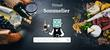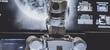# Detect text toxicity using React

In a previous article I explained how to start with Tensorflow.js, from creating a simple linear regression model to using pretrained models as posenet. I highly recommend to read this article to understand how it works.

In today's article I want to show you how easy it's to use the text toxicity detection model without any previous knowledge of machine learning.

## What is text toxicity detection?

Toxicity detection detects text containing toxic content such as threatening language, insults, obscenities, identity-based hate, or sexually explicit language.Fig 1: Text tocicity example

With this in your browser, it'll be esier to prevent unwanted comments/opinions and speed up the review process of these content.

However, this looks so complicated... Nah, good news for you! You don't need to be a machine-learning expert to use this model. Let's see how.

## The hook

I wrote a React hook to simplify the way to use it, so you can get the predictions of text just by using a hook in one line of code:

``````import useTextToxicity from 'react-text-toxicity'

// Usage inside your component or custom hook
const predictions = useTextToxicity('This is an example')
/*
{
"label": "identity_attack",
"match": false,
"probability": "3.40%",
"probabilities": [0.9659664034843445, 0.03403361141681671],
},
{
"label": "insult",
"match": true,
"probability": "91.8%",
"probabilities": [0.08124706149101257, 0.9187529683113098],
},
...
*/
``````

I uploaded the npm package so you can use it by doing:

``````yarn add react-text-toxicity
``````

And the GitHub repo 👉 https://github.com/aralroca/react-text-toxicity

We can connect the `useTextToxicity` hook to a `state` by using:

``````const [value, setValue] = useState('')
const predictions = useTextToxicity(value)

//...
;<textarea value={value} onChange={(e) => setValue(e.target.value)} />
``````

This way, everytime that the value changes, the predictions will be updated (we can predict "on the fly").

Here's the full example code of Fig 1:

``````import React, { Fragment, useState } from 'react'
import useTextToxicity from 'react-text-toxicity'

function Toxicity({ predictions }) {
const style = { margin: 10 }

return (
<div style={style}>
{predictions.map(({ label, match, probability }) => (
<div style={{ margin: 5 }} key={label}>
{`\${label} - \${probability} - \${match ? '🤢' : '🥰'}`}
</div>
))}
</div>
)
}

export default function Index() {
const [value, setValue] = useState('')

// predictions are updated every time the value is updated
const predictions = useTextToxicity(value)

return (
<div style={{ display: 'flex' }}>
<div>
<div>Write something</div>
<textarea
style={{ width: 300, height: 200 }}
value={value}
onChange={(e) => setValue(e.target.value)}
/>
</div>
{value && <Toxicity predictions={predictions} />}
</div>
)
}
``````

## Under the "hook"

Under the hood, the hook is using Tensorflow.js toxicity model:

If you need to implement the same outside React, you can use this repo.

## Conclusion

Sometimes when we listen about machine learning and/or Tensorflow our mind disconnects, we think that's too complicated for us. However, there are pretrained models we can use without headaches.

The usage of React hooks facilitates data prediction from pretrained models in one simple line of code.

Now, I encourage you to experiment with these Tensorflow models or start using them in your projects!

More...## Image classifier: in the browser